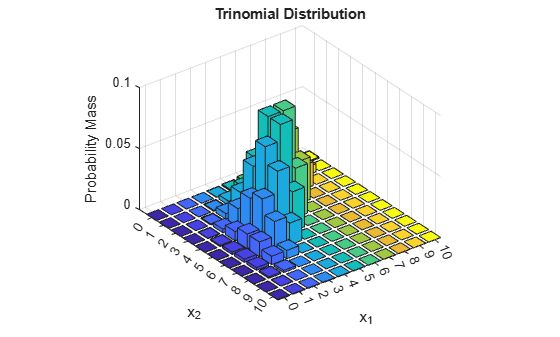mnpdf

Multinomial probability density function

Syntax

Y = mnpdf(X,PROB)

Description

Y = mnpdf(X,PROB) returns the pdf for the multinomial distribution with probabilities PROB, evaluated at each row of X. X and PROB are m-by-k matrices or 1-by-k vectors, where k is the number of multinomial bins or categories. Each row of PROB must sum to one, and the sample sizes for each observation (rows of X) are given by the row sums sum(X,2). Y is an m-by-1 vector, and mnpdf computes each row of Y using the corresponding rows of the inputs, or replicates them if needed.

Examples

collapse all

Compute the pdf of a multinomial distribution with a sample size of n = 10. The probabilities are p = 1/2 for outcome 1, p = 1/3 for outcome 2, and p = 1/6 for outcome 3.

p = [1/2 1/3 1/6];
n = 10;
x1 = 0:n;
x2 = 0:n;
[X1,X2] = meshgrid(x1,x2);
X3 = n-(X1+X2);

Compute the pdf of the distribution.

Y = mnpdf([X1(:),X2(:),X3(:)],repmat(p,(n+1)^2,1));

Plot the pdf on a 3-dimensional figure.

Y = reshape(Y,n+1,n+1);
bar3(Y)
h = gca;
h.XTickLabel = [0:n];
h.YTickLabel = [0:n];
xlabel('x_1')
ylabel('x_2')
zlabel('Probability Mass')
title('Trinomial Distribution')Note that the visualization does not show x3, which is determined by the constraint x1 + x2 + x3 = n.